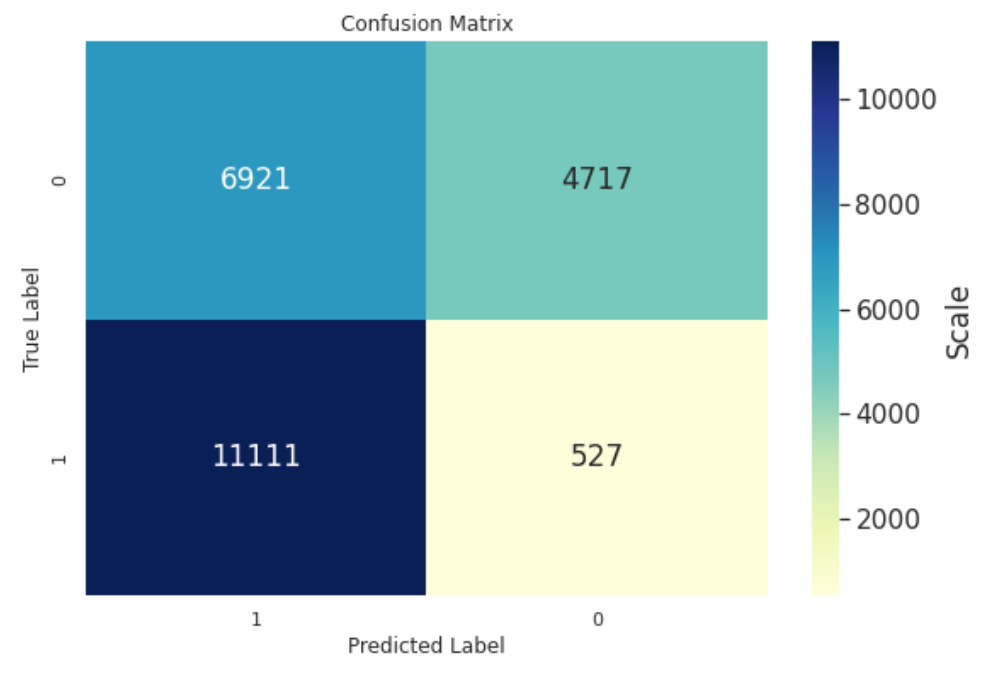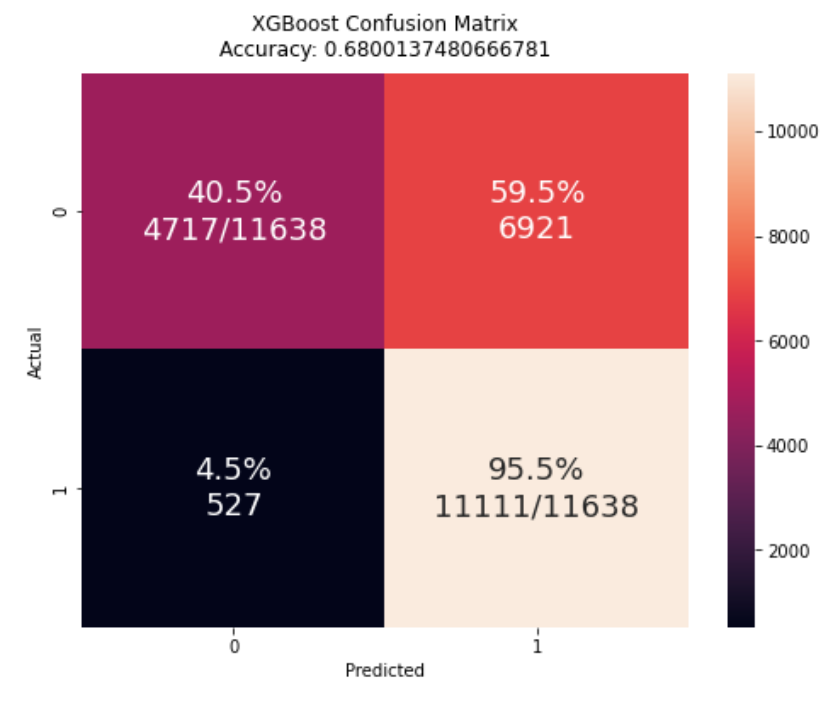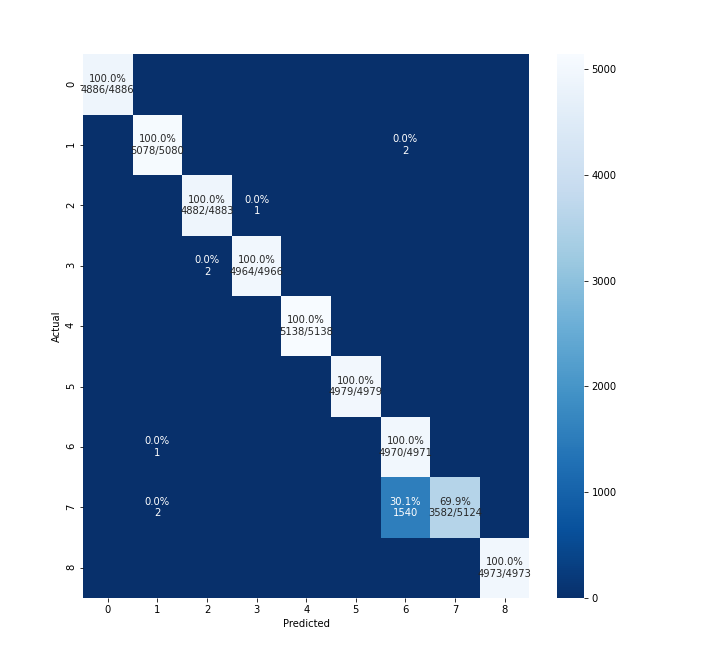Instantly share code, notes, and snippets.

# hitvoice/plot_confusion_matrix.png

Last active June 15, 2023 12:39
Star You must be signed in to star a gist
Generate matrix plot for confusion matrix with pretty annotations.This file contains bidirectional Unicode text that may be interpreted or compiled differently than what appears below. To review, open the file in an editor that reveals hidden Unicode characters. Learn more about bidirectional Unicode characters
 import numpy as np import pandas as pd import matplotlib.pyplot as plt import seaborn as sns from sklearn.metrics import confusion_matrix def cm_analysis(y_true, y_pred, filename, labels, ymap=None, figsize=(10,10)): """ Generate matrix plot of confusion matrix with pretty annotations. The plot image is saved to disk. args: y_true: true label of the data, with shape (nsamples,) y_pred: prediction of the data, with shape (nsamples,) filename: filename of figure file to save labels: string array, name the order of class labels in the confusion matrix. use `clf.classes_` if using scikit-learn models. with shape (nclass,). ymap: dict: any -> string, length == nclass. if not None, map the labels & ys to more understandable strings. Caution: original y_true, y_pred and labels must align. figsize: the size of the figure plotted. """ if ymap is not None: y_pred = [ymap[yi] for yi in y_pred] y_true = [ymap[yi] for yi in y_true] labels = [ymap[yi] for yi in labels] cm = confusion_matrix(y_true, y_pred, labels=labels) cm_sum = np.sum(cm, axis=1, keepdims=True) cm_perc = cm / cm_sum.astype(float) * 100 annot = np.empty_like(cm).astype(str) nrows, ncols = cm.shape for i in range(nrows): for j in range(ncols): c = cm[i, j] p = cm_perc[i, j] if i == j: s = cm_sum[i] annot[i, j] = '%.1f%%\n%d/%d' % (p, c, s) elif c == 0: annot[i, j] = '' else: annot[i, j] = '%.1f%%\n%d' % (p, c) cm = pd.DataFrame(cm, index=labels, columns=labels) cm.index.name = 'Actual' cm.columns.name = 'Predicted' fig, ax = plt.subplots(figsize=figsize) sns.heatmap(cm, annot=annot, fmt='', ax=ax) plt.savefig(filename)

### koursera commented Oct 5, 2020

Hi Hitvoice - this is awesome! Thanks so much posting this. I'm trying it out and see an odd thing with my binary classification CM. I've posted your version and what I get from using confusion_matrix. You can see that the classes are transposed and I'm wondering if I'm doing something wrong. I'd like to keep this aligned to what confusion_matrix displays so I thought I'd ask.### hitvoice commented Oct 28, 2020

Hi Hitvoice - this is awesome! Thanks so much posting this. I'm trying it out and see an odd thing with my binary classification CM. I've posted your version and what I get from using confusion_matrix. You can see that the classes are transposed and I'm wondering if I'm doing something wrong. I'd like to keep this aligned to what confusion_matrix displays so I thought I'd ask.What do you mean by "the classes are transposed"? Do you mean the order of 0 and 1?

### koursera commented Nov 3, 2020

Do you mean the order of 0 and 1?

Yes, I was looking to align this with sklearn's confusion matrix for consistency. Perhaps a parameter could be passed to indicate the order since various references have a different convention for the axes.

### hitvoice commented Nov 4, 2020

You can see from the code that the matrix is indeed computed from sklearn's "confusion_matrix" function. How did you get the first figure? If you prefer that kind of style, you can reorder the dataframe columns by `cm = cm[cm.columns[::-1]]` before creating the plot.

### kalkite commented Oct 11, 2021 • edited

@hitvoice, it's really appreciated work. how to changet the `clf.classes_` to other, for instance my classess are from 0 to 8, but i want to change those labels to string.### hitvoice commented Oct 17, 2021

@rajeshkalakoti pass the value of `ymap` (dict[Any,str]) to `cm_analysis`. You can configure the class names in `ymap`.

### kalkite commented Oct 24, 2021

for instance, labels are like "class-1, class-2,class-3, class-4,class-5, class-6,class-7, class-8,classs-9", how to configure it?